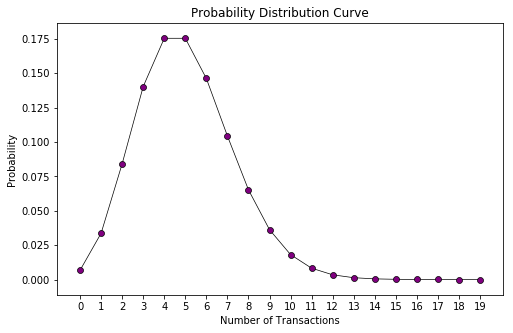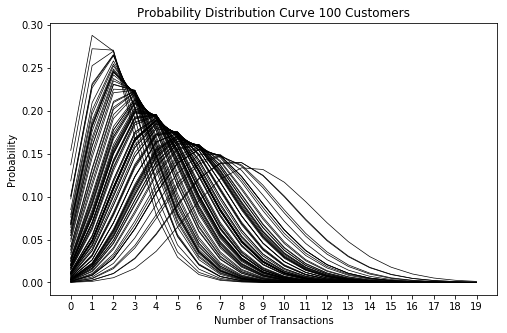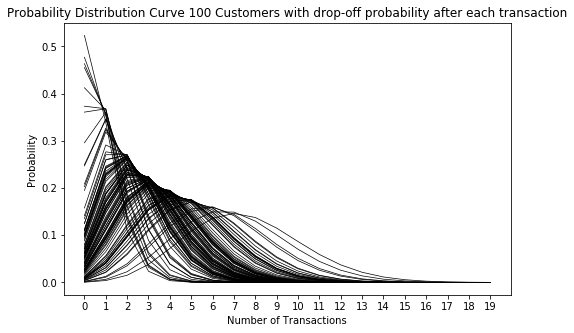## BG-NBD Model for Customer Base Analysis in Python

• Post author:
• Post category:Python

# BG-NBD Model for Customer Base Analysis

### Introduction

In this post we will use python to replicate the BG-NBD (Beta Geometric Negative Binomial Distribution) model that is described in the paper “Counting Your Customers” the Easy Way: An Alternative to the Pareto/NBD Model by Fader et al. in 2005.

We will use the Excel worksheet that was explored in the original research paper, which can be found here.

The model can be used to determine the expected repeat visits for customers in order to determine a customers lifetime value. It can also be used to determine whether a customer has churned or is likely to churn soon.

The model is already implemented in python in the lifetimes package from Cameron Davidson Pilon at Shopify but this post aims to break this open and explore the steps we go through when using this model.

### Assumptions

This model has a number of assumptions associated with it. These are as follows:

1. While active, transactions made by a customer in time period $t$ is Poisson distributed with mean $\lambda t$

Let’s look at the probability distribution of one customer with $\lambda = 5$:

In :
import matplotlib.pyplot as plt
from scipy.stats import poisson

probability_arr = []
distribution = poisson(5)
for transactions in range(0,20):
probability_arr.append(distribution.pmf(transactions))

plt.figure(figsize=(8,5))
plt.ylabel('Probability')
plt.xlabel('Number of Transactions')
plt.xticks(range(0, 20))
plt.title('Probability Distribution Curve')
plt.plot(probability_arr, color='black', linewidth=0.7, zorder=1)
plt.scatter(range(0, 20), probability_arr, color='purple', edgecolor='black', linewidth=0.7, zorder=2)
plt.show()2. Differences in transaction rate between customers follows a gamma distribution with shape $r$ and scale $\alpha$

Let’s take a look at how the probability distributions would look for 100 customers with $r=9.0$ and $\alpha=0.5$:

In :
import numpy as np

plt.figure(figsize=(8,5))

for customer in range(0, 100):
distribution = poisson(np.random.gamma(shape=9, scale=0.5))
probability_arr = []
for transactions in range(0,20):
probability_arr.append(distribution.pmf(transactions))
plt.plot(probability_arr, color='black', linewidth=0.7, zorder=1)

plt.ylabel('Probability')
plt.xlabel('Number of Transactions')
plt.xticks(range(0, 20))
plt.title('Probability Distribution Curve 100 Customers')

plt.show()3. Each customer becomes inactive after each transaction with probability $p$

4. Differences in $p$ follows a beta distribution with shape parameters $a$ and $b$

Let’s apply a random drop-off with probability $p$ that is beta distributed with parameters $a=1.0$ and $b=2.5$ to each of our 100 customers after each transaction and see what this does to our probability distribution curves:

In :
import numpy as np

plt.figure(figsize=(8,5))

for customer in range(0, 100):
distribution = poisson(np.random.gamma(shape=9, scale=0.5))
probability_arr = []
beta = np.random.beta(a=1.0, b=2.5)
cumulative_beta = 0
for transactions in range(0,20):
proba = distribution.pmf(transactions)
cumulative_beta = beta + cumulative_beta - (beta * cumulative_beta)
inactive_probability = 1 - cumulative_beta
proba *= inactive_probability
probability_arr.append(proba)
probability_arr = np.array(probability_arr)
probability_arr /= probability_arr.sum()
plt.plot(probability_arr, color='black', linewidth=0.7, zorder=1)

plt.ylabel('Probability')
plt.xlabel('Number of Transactions')
plt.xticks(range(0, 20))
plt.title('Probability Distribution Curve 100 Customers with drop-off probability after each transaction')

plt.show()We can see that this transformation moves the distribution to the left. This makes sense as there is an increased likelihood of fewer transactions because after each transaction there is the probability that the customer doesn’t return and this accumulates with each additional transaction.

5. Transaction rate and dropout probability vary independently between customers

Let’s start by loading in the raw data from the Excel file into a pandas DataFrame.

In :
import pandas as pd


Out:
x t_x T
ID
1 2 30.428571 38.857143
2 1 1.714286 38.857143
3 0 0.000000 38.857143
4 0 0.000000 38.857143
5 0 0.000000 38.857143

In this dataset:

$x$ is the number of repeat purchases from this customer since the first purchase (frequency)
$t_x$ is the date of the most recent purchase in weeks since the customer’s first purchase (recency)
$T$ is the time to be considered in weeks since the customer’s first purchase

### Optimising likelihood function parameters

Given the above assumptions, the likelihood a customer makes $x$ purchases in a given time period $T$ is:

$L(\lambda, p | X=x, T) = (1-p)^x \lambda^x e^{-\lambda T} + \delta_{x>0}p(1-p)^{x-1}\lambda^x e^{-\lambda t_x}$

However, this depends on knowing the variables $\lambda$ and $p$, which are both unobserved quantities.

We can, instead, write the likelihood function of a randomly-chosen individual with purchase history $X=x, t_x, T$ as:

$L(r, \alpha, a, b|X=x, t_x, T) = A_1 A_2 (A_3 + \delta_{x>0} A_4)$

Where:

$A_1 = \dfrac{\Gamma(r+x)\alpha^r}{\Gamma(r)}$

$A_2 = \dfrac{\Gamma(a+b)\Gamma(b+x)}{\Gamma(b) + \Gamma(a+b+x)}$

$A_3 = \dfrac{1}{\alpha + T}^{r+x}$

$A_4 = \bigg( \dfrac{a}{b+x-1}\bigg)\bigg(\dfrac{1}{\alpha + t_x}\bigg)^{r+x}$

We can then optimise the paramaters of the likelihood function by optimising the log-likelihood function, such that the log-likelihood function is:

$\ln[L(r, \alpha, a, b|X=x, t_x, T) = \ln(A_1) \ln(A_2) \ln(e^{\ln(A_3)} + \delta_{x>0} e^{\ln(A_4)})]$

Where:

$\ln(A_1) = \ln[\Gamma(r+x)] – \ln[\Gamma(r)] + r\ln(\alpha)$

$\ln(A_2) = \ln[\Gamma(a+b)] + \ln[\Gamma(b+x)] – \ln[\Gamma(b)] – \ln[\Gamma(a+b+x)]$

$\ln(A_3) = -(r+x) \ln(\alpha + T)$

$\ln(A_4) = \begin{cases} \ln(a) – \ln(b+x-1) – (r+x)\ln(\alpha + t_x) & \text{if}\ x>0 \\ 0 & \text{otherwise} \end{cases}$

Let’s define this as a negative log-likelihood in python:

In :
from scipy.special import gammaln

def negative_log_likelihood(params, x, t_x, T):
if np.any(np.asarray(params) <= 0):
return np.inf

r, alpha, a, b = params

ln_A_1 = gammaln(r + x) - gammaln(r) + r * np.log(alpha)
ln_A_2 = (gammaln(a + b) + gammaln(b + x) - gammaln(b) -
gammaln(a + b + x))
ln_A_3 = -(r + x) * np.log(alpha + T)
ln_A_4 = x.copy()
ln_A_4[ln_A_4 > 0] = (
np.log(a) -
np.log(b + ln_A_4[ln_A_4 > 0] - 1) -
(r + ln_A_4[ln_A_4 > 0]) * np.log(alpha + t_x)
)

delta =  np.where(x>0, 1, 0)

log_likelihood = ln_A_1 + ln_A_2 + np.log(np.exp(ln_A_3) + delta * np.exp(ln_A_4))
return -log_likelihood.sum()


Now we can optimise our parameters. We’ll use the Nelder-Mead Simplex algorithm, which is a heuristic, non-gradient search method to minimise our negative log likelihood cost function.

In :
from scipy.optimize import minimize

scale = 1 / df['T'].max()
scaled_recency = df['t_x'] * scale
scaled_T = df['T'] * scale

def _func_caller(params, func_args, function):
return function(params, *func_args)

current_init_params = np.array([1.0, 1.0, 1.0, 1.0])
output = minimize(
_func_caller,
tol=0.0001,
x0=current_init_params,
args=([df['x'], scaled_recency, scaled_T], negative_log_likelihood),
options={'maxiter': 2000}
)

r = output.x
alpha = output.x
a = output.x
b = output.x

alpha /= scale

print("r = {}".format(r))
print("alpha = {}".format(alpha))
print("a = {}".format(a))
print("b = {}".format(b))

r = 0.242594123569
alpha = 4.41358813135
a = 0.792935471652
b = 2.42595536972


### Expected Sales Forecasting

So now that we have our optimised parameters $r$, $\alpha$, $a$ and $b$, we can use these to compute our repeat sales forecast for our cohort of customers across time period of length $t$ using the following formula for any given individual:

$E(X(t)|r, \alpha, a, b) = \dfrac{a+b-1}{a-1}\bigg[ 1 – \bigg(\dfrac{\alpha}{\alpha + t}\bigg)^r {}_{2}F_{1}(r, b; a+b-1;\dfrac{t}{\alpha+t}) \bigg]$

Where ${}_{2}F_{1}$ is the Gaussian hypergeometric function, which takes the form:

${}_{2}F_{1}(a, b; c; z) = \sum\limits_{j=0}^{\infty} u_j$

$\text{where } u_j = \dfrac{(a)_j(b)_j}{(c)_j)}\dfrac{z^j}{j!}$

This can be expanded to the series:

$\dfrac{u_j}{u_{j-1}} = \dfrac{(a+j-1)(b+j-1)}{(c+j-1)j}z$

And we can substitute the values above for these $a$, $b$, $c$ and $z$ values of ${}_{2}F_{1}$

In :
from scipy.special import hyp2f1

def expected_sales_to_time_t(t):
hyp2f1_a = r
hyp2f1_b = b
hyp2f1_c = a + b - 1
hyp2f1_z = t / (alpha + t)
hyp_term = hyp2f1(hyp2f1_a, hyp2f1_b, hyp2f1_c, hyp2f1_z)

return ((a + b - 1) / (a - 1)) * (1-(((alpha / (alpha+t)) ** r) * hyp_term))


So now we can test out our expectes sales for any given individual in our cohort, let’s say we want to see how many purchases we can expect from an individual across a period of one year (52 weeks):

In :
expected_sales_to_time_t(52)

Out:
1.444010643699092

So we would expect 1.44 sales for any given individual across the next year.

Now will calculate the cumulative repeat sales for 78 weeks from our cohort of customers.

To calculate cumulative repeat sales for a forecast across our cohort, we can’t just scale up to number of customers, we need to take into account the fact that each customer had a different time of first purchase.

$n_s$ is the number of people that had their first purchase on day $s$

If $S$ is the total possible number of start dates:

$\text{Total repeat transactions to } t = \sum\limits_{s=0}^{S} \delta_{(t>s)} n_s E[X(t-s)]$

where:

$\delta_{(t>s)} = \begin{cases} 1 & \text{if}\ t>s \\ 0 & \text{otherwise} \end{cases}$

In :
# Period of consideration is 39 weeks.
# T indicates the length of time since first purchase
n_s = (39 - df['T']).value_counts().sort_index()


Out:
0.142857    18
0.285714    22
0.428571    17
0.571429    20
0.714286    23
Name: T, dtype: int64

18 people made their first transaction on day 1 (1/7 weeks), 22 on day 2 (2/7 weeks), 17 on day 3 (1/7 weeks) etc.

In :
forecast_range = np.arange(0, 78, 1/7.0)

def cumulative_repeat_transactions_to_t(t):
expected_transactions_per_customer = (t - n_s.index).map(lambda x: expected_sales_to_time_t(x) if x > 0 else 0)
expected_transactions_all_customers = (expected_transactions_per_customer * n_s).values
return expected_transactions_all_customers.sum()

cum_rpt_sales = pd.Series(map(cumulative_repeat_transactions_to_t, forecast_range), index=forecast_range)

cum_rpt_sales.tail(10)

Out:
76.571429    4109.744742
76.714286    4114.856053
76.857143    4119.961614
77.000000    4125.061441
77.142857    4130.155549
77.285714    4135.243956
77.428571    4140.326675
77.571429    4145.403724
77.714286    4150.475118
77.857143    4155.540873
dtype: float64

So across the next 78 weeks we would expect to make around 4156 repeat transactions from our cohort of customers

### Conditional Expectation

If we want to calculate the expected sales from a single customer (how many transacations any single customer will make going forward in time period $t$), we can calculate the conditional expectation for that customer.

The expression used to calculate this is:

$E(Y(t)|X=x, t_x, T, r, \alpha, a, b) = \dfrac{a + b + x – 1}{a-1} \times \dfrac{\bigg[1 – \bigg(\dfrac{\alpha + T}{\alpha + T + t}\bigg)^{r+x}{}_{2}F_{1}(r+x, b+x; a+b+x-1; \dfrac{t}{\alpha+T+t})\bigg]}{1 + \delta_{(x>0)}\dfrac{a}{b+x-1}\bigg(\dfrac{\alpha + T}{\alpha + t_x}\bigg)^{r+x}}$

In :
def calculate_conditional_expectation(t, x, t_x, T):
first_term = (a + b + x - 1) / (a-1)
hyp2f1_a = r + x
hyp2f1_b = b + x
hyp2f1_c = a + b + x - 1
hyp2f1_z = t / (alpha + T + t)
hyp_term = hyp2f1(hyp2f1_a, hyp2f1_b, hyp2f1_c, hyp2f1_z)
second_term = (1 - ((alpha + T) / (alpha + T + t)) ** (r + x) * hyp_term)
delta = 1 if x > 0 else 0
denominator = 1 + delta * (a / (b + x - 1)) * ((alpha + T) / (alpha + t_x)) ** (r+x)
return first_term * second_term / denominator

In :
calculate_conditional_expectation(39, 2, 30.43, 38.86)

Out:
1.225904664486748

So we would expect a customer with:

$x = 2$
$t_x = 30.43$
$T = 38.86$

to make 1.22 purchases over the next 39 weeks, given that they’re drawn from the same cohort as the others used to calculate our parameters.